Courses

Simple Harmonic Motion MCQ

10 Questions MCQ Test Topic wise Tests for IIT JAM Physics | Simple Harmonic Motion MCQ

Description
Attempt Simple Harmonic Motion MCQ | 10 questions in 30 minutes | Mock test for IIT JAM preparation | Free important questions MCQ to study Topic wise Tests for IIT JAM Physics for IIT JAM Exam | Download free PDF with solutions
QUESTION: 1

The equation of S.H.M of a particle is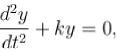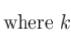is a positive constant. The time period of motion is given by :

Solution:

Compare with equation of SHM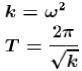The correct answer is: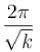QUESTION: 2

If length of simple pendulum is increased by 6 % then percentage change in time period will be :

Solution: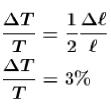The correct answer is: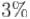QUESTION: 3

A particle is executing S.H.M. from mean position at 5 cm distance, acceleration is 20 cm/s2 then value of angular velocity will be :

Solution: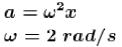QUESTION: 4

A man measures the period of a simple pendulum inside a stationary lift and finds it to be T sec. If the lift accelerates upwards with an acceleration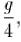then the period of the pendulum will be :

Solution: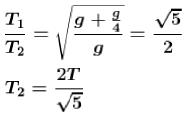The correct answer is: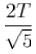QUESTION: 5

The maximum velocity of harmonic oscillator is a, and its maximum acceleration is β Its time period will be :

Solution: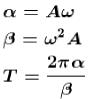The correct answer is: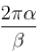QUESTION: 6

One mass m is suspended from a spring. Time period of oscillation is T. Now if spring is divided into n piece and these are joined in parallel order then time period of oscillation if same mass is suspended.

Solution:

Keq = n2k is correct but it is a 2 step process
First, K’ = k x n as the spring constant becomes ‘n’ times due to shortening of length
Second, Keq = n x K’ as they are connected in a parallel manner
=>Keq = n2K

QUESTION: 7

Amplitude of harmonic oscillator is a when velocity of particle is half of maximum velocity, then position of particle will be :

Solution: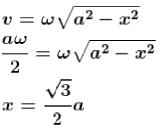The correct answer is: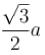QUESTION: 8

A simple pendulum suspended from the ceiling of a stationary trolley has a length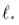Its period of oscillation is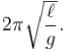What will be its period of oscillation if the trolley moves forward with an acceleration f ?

Solution: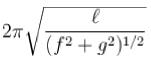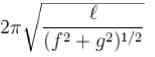QUESTION: 9

In S.H.M, the graph between kinetic energy 'K' and time 't' is:

Solution: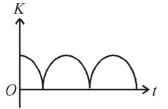QUESTION: 10

Two object A and B of equal mass are suspended from two springs of spring constants KA and KB if the objects oscillate vertically in such a manner that their maximum kinetic energies are equal, then the ratio of their amplitude is :

Solution:

Kinetic energy of A = Kinetic energy of B.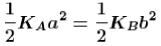So, ratio of amplitude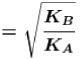The correct answer is: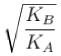Use Code STAYHOME200 and get INR 200 additional OFF Use Coupon Code

Track your progress, build streaks, highlight & save important lessons and more!

Similar ContentRelated tests